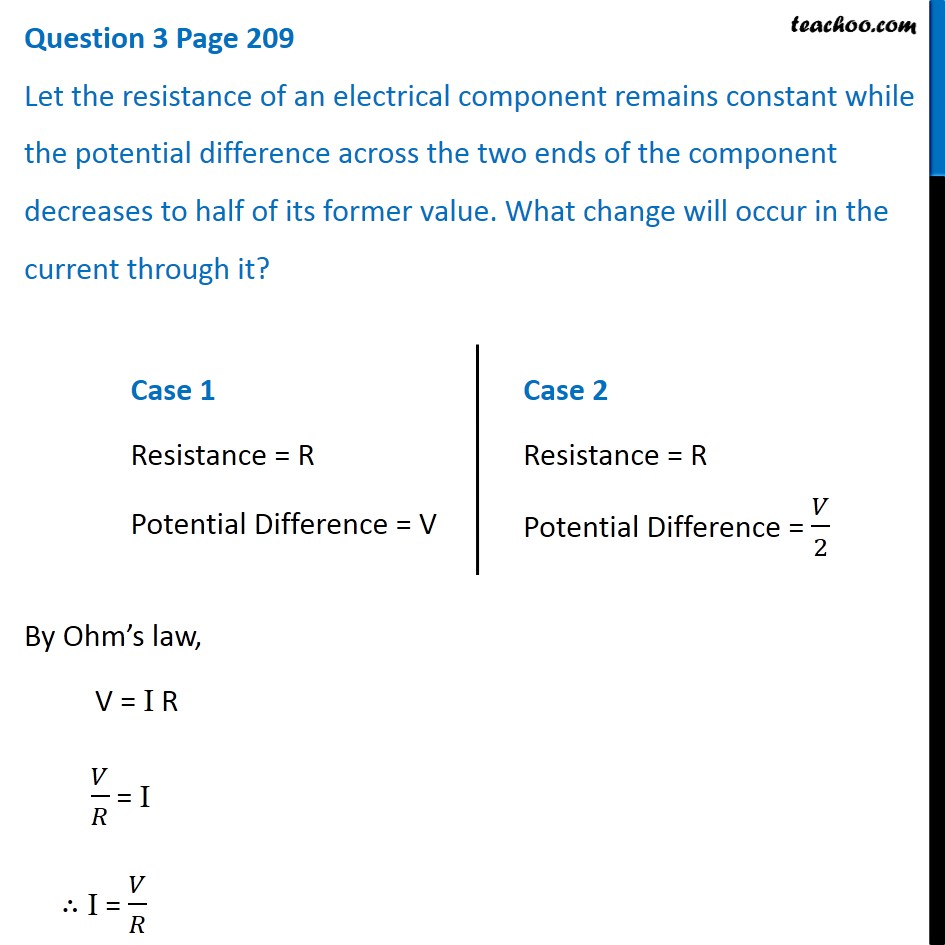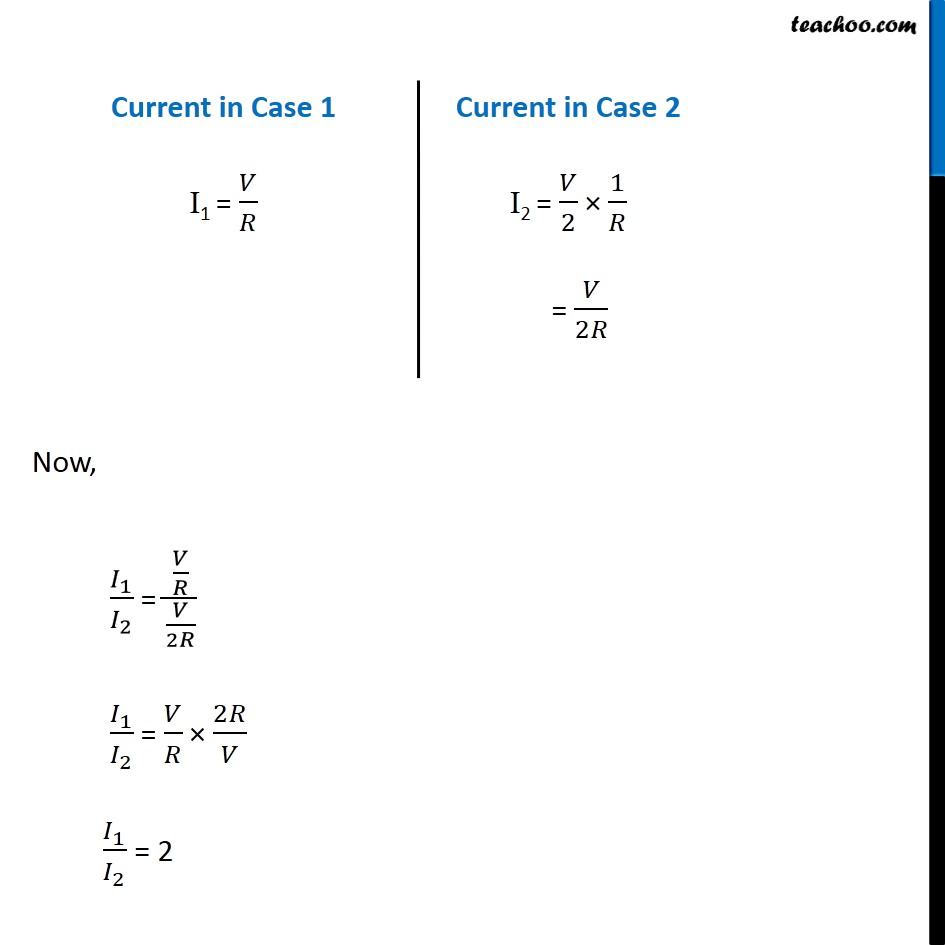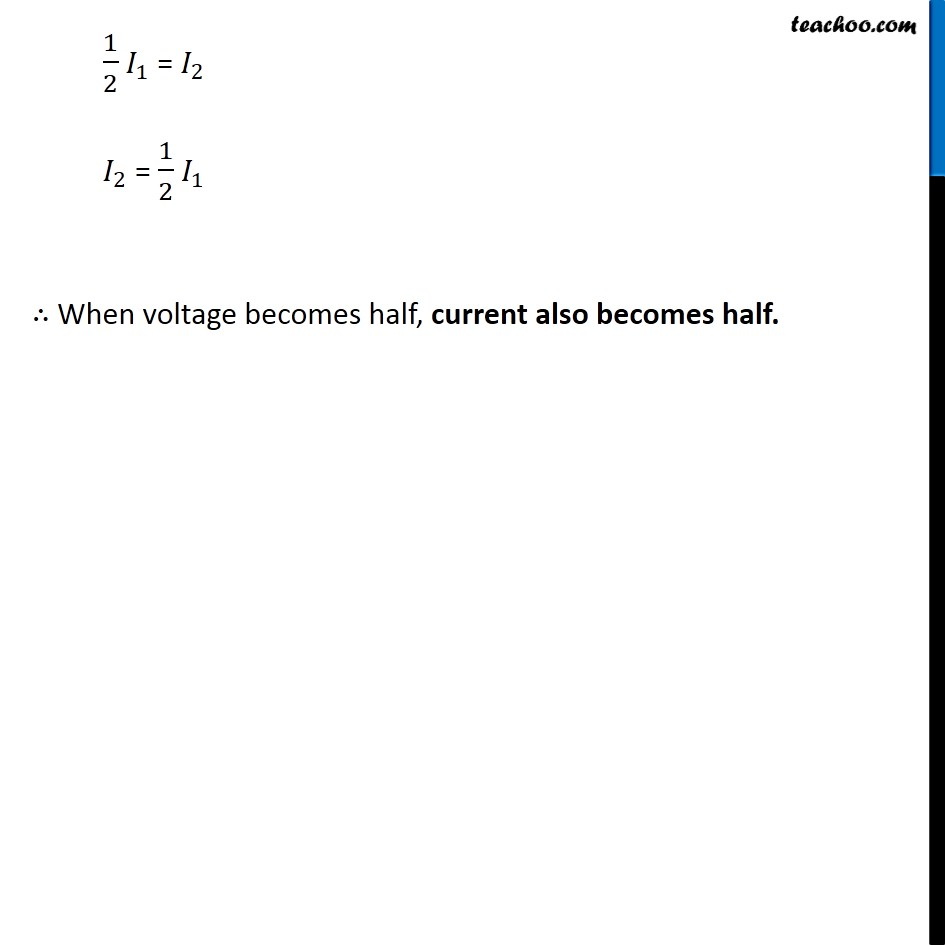Questions from Inside the chapter

Class 10
Chapter 12 Class 10 - Electricity

## Let the resistance of an electrical component remains constant while the potential difference across the two ends of the component decreases to half of its former value. What change will occur in the current through it?Learn in your speed, with individual attention - Teachoo Maths 1-on-1 Class

### Transcript

Case 1 Resistance = R Potential Difference = V Case 2 Resistance = R Potential Difference = 𝑉/2 By Ohm’s law, V = I R 𝑉/𝑅 = I ∴ I = 𝑉/𝑅 Current in Case 1 I1 = 𝑉/𝑅 Current in Case 2 I2 = 𝑉/2 × 1/𝑅 = 𝑉/2𝑅 Now, 𝐼_1/𝐼_2 = (𝑉/𝑅)/(𝑉/2𝑅) 𝐼_1/𝐼_2 = 𝑉/𝑅 × 2𝑅/𝑉 𝐼_1/𝐼_2 = 2 1/2 𝐼_1 = 𝐼_2 𝐼_2 = 1/2 𝐼_1 ∴ When voltage becomes half, current also becomes half.